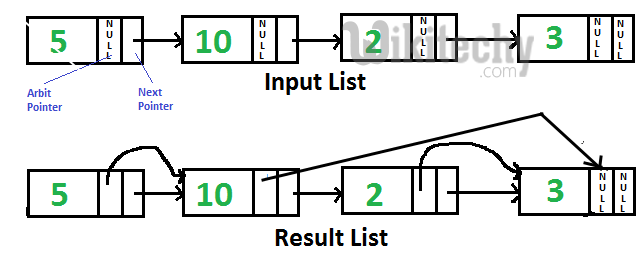# Cpp Algorithm-Point arbit pointer to greatest value right side node in a linked list

Cpp Algorithm-Point arbit pointer to greatest value right side node in a linked list-Linked list Given singly linked list with every node

Given singly linked list with every node having an additional “arbitrary” pointer that currently points to NULL. We need to make the “arbitrary” pointer to greatest value node in a linked list on its right side.A Simple Solution is to traverse all nodes one by one. For every node, find the node which has greatest value on right side and change the next pointer. Time Complexity of this solution is O(n2).

An Efficient Solution can work in O(n) time. Below are steps.

1. Reverse given linked list.
2. Start traversing linked list and store maximum value node encountered so far. Make arbit of every node to point to max. If the data in current node is more than max node so far, update max.
3. Reverse modified linked list and return head.

C++ Programming:

``````// C++ program to point arbit pointers to highest
// value on its right
#include<bits/stdc++.h>
using namespace std;

/* Link list node */
struct Node
{
int data;
Node* next, *arbit;
};

/* Function to reverse the linked list */
Node* reverse(Node *head)
{
Node *prev = NULL, *current = head, *next;
while (current != NULL)
{
next  = current->next;
current->next = prev;
prev = current;
current = next;
}
return prev;
}

// This function populates arbit pointer in every
// node to the greatest value to its right.
Node* populateArbit(Node *head)
{
// Reverse given linked list
head = reverse(head);

// Initialize pointer to maximum value node
Node *max = head;

// Traverse the reversed list
Node *temp = head->next;
while (temp != NULL)
{
// Connect max through arbit pointer
temp->arbit = max;

// Update max if required
if (max->data < temp->data)
max = temp;

// Move ahead in reversed list
temp = temp->next;
}

// Reverse modified linked list and return
// head.
return reverse(head);
}

// Utility function to print result linked list
void printNextArbitPointers(Node *node)
{
printf("Node\tNext Pointer\tArbit Pointer\n");
while (node!=NULL)
{
cout << node->data << "\t\t";

if (node->next)
cout << node->next->data << "\t\t";
else cout << "NULL" << "\t\t";

if (node->arbit)
cout << node->arbit->data;
else cout << "NULL";

cout << endl;
node = node->next;
}
}

/* Function to create a new node with given data */
Node *newNode(int data)
{
Node *new_node = new Node;
new_node->data = data;
new_node->next = NULL;
return new_node;
}

/* Driver program to test above functions*/
int main()
{
Node *head = newNode(5);
head->next = newNode(10);
head->next->next = newNode(2);
head->next->next->next = newNode(3);

head = populateArbit(head);

printf("Resultant Linked List is: \n");
printNextArbitPointers(head);

return 0;
}``````

Output:

```Resultant Linked List is:
Node    Next Pointer    Arbit Pointer
5               10              10
10              2               3
2               3               3
3               NULL            NULL```

Recursive Solution:

READ  Python Algorithm - Pairwise swap elements of a given linked list

We can recursively reach the last node and traverse the linked list from end. Recursive solution doesn’t require reversing of linked list. We can also use a stack in place of recursion to temporarily hold nodes. Thanks to Santosh Kumar Mishra for providing this solution.

C++ Programming:

``````// C++ program to point arbit pointers to highest
// value on its right
#include<bits/stdc++.h>
using namespace std;

/* Link list node */
struct Node
{
int data;
Node* next, *arbit;
};

// This function populates arbit pointer in every
// node to the greatest value to its right.
void populateArbit(Node *head)
{
// using static maxNode to keep track of maximum
// orbit node address on right side
static Node *maxNode;

// if head is null simply return the list
if (head == NULL)
return;

/* if head->next is null it means we reached at
the last node just update the max and maxNode */
if (head->next == NULL)
{
maxNode = head;
return;
}

/* Calling the populateArbit to the next node */
populateArbit(head->next);

/* updating the arbit node of the current
node with the maximum value on the right side */
head->arbit = maxNode;

/* if current Node value id greater then
the previous right node then  update it */
if (head->data > maxNode->data)
maxNode = head;

return;
}

// Utility function to print result linked list
void printNextArbitPointers(Node *node)
{
printf("Node\tNext Pointer\tArbit Pointer\n");
while (node!=NULL)
{
cout << node->data << "\t\t";

if(node->next)
cout << node->next->data << "\t\t";
else cout << "NULL" << "\t\t";

if(node->arbit)
cout << node->arbit->data;
else cout << "NULL";

cout << endl;
node = node->next;
}
}

/* Function to create a new node with given data */
Node *newNode(int data)
{
Node *new_node = new Node;
new_node->data = data;
new_node->next = NULL;
return new_node;
}

/* Driver program to test above functions*/
int main()
{
Node *head = newNode(5);
head->next = newNode(10);
head->next->next = newNode(2);
head->next->next->next = newNode(3);

populateArbit(head);

printf("Resultant Linked List is: \n");
printNextArbitPointers(head);

return 0;
}``````

Output:

```Resultant Linked List is:
Node    Next Pointer    Arbit Pointer
5               10              10
10              2               3
2               3               3
3               NULL            NULL```

#### About the author#### Venkatesan Prabu

Wikitechy Founder, Author, International Speaker, and Job Consultant. My role as the CEO of Wikitechy, I help businesses build their next generation digital platforms and help with their product innovation and growth strategy. I'm a frequent speaker at tech conferences and events.

X